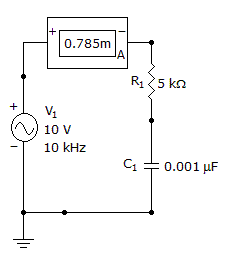# Electronics - Capacitors - Discussion

### Discussion :: Capacitors - General Questions (Q.No.54)

54.

What is the reactive power?[A]. 6.8 mW [B]. 9.8 mW [C]. 12.8 mW [D]. 15.8 mW

Explanation:

No answer description available for this question.

 Saranya said: (Sep 22, 2011) Can anyone explain this..?

 Saranya said: (Sep 22, 2011) Can anyone tell me what is the formula used for this ?

 Vasu said: (Sep 27, 2011) The formula being used here is Power =I^2 * Xc = (0.785mA)^2 * (1/WC) = (0.785 mA)^2 * (1/ (2*3.14 *Freq * Capacitance) =9.8 mWatts

 San said: (Apr 10, 2013) To find reactive power Pr = I^2*Xc. Where Xc = 1/wc, w = 2pif. XC = 1/2*PI*fc. = 1/6.28*10000*0.001*E-6. = 15923.5 ohms. Pr = I^2*Xc. = 0.785^2*15923.5. = 9812.4W. = 9.8mW.

 Pritee said: (Oct 4, 2013) The same is calculated by formula, Pr=v*v/Xc.

 Ghufran said: (Nov 4, 2014) VAR or Watts?

 Lex said: (Jun 18, 2018) 9812.4 Watts is not 9.8mW. It should be kW.

 Chuchu said: (Sep 20, 2018) If you're going to use V^2/Xc, you must first find the voltage in the capacitor since the circuit is in series. So it is more reliable to use I^2 * Xc in the given.

 Hani said: (Mar 19, 2019) P = iv cos (θ). θ= tan(inverse)xc\R.

 Kal said: (Oct 13, 2019) It's 0.785 mA. So mW, not kW.

 Mayuresh said: (Mar 29, 2020) Please let me explain the formula.